## CPU Scheduling Algorithms-

Various CPU scheduling algorithms are-

## Problem-01:

Consider three process, all arriving at time zero, with total execution time of 10, 20 and 30 units respectively. Each process spends the first 20% of execution time doing I/O, the next 70% of time doing computation, and the last 10% of time doing I/O again. The operating system uses a shortest remaining compute time first scheduling algorithm and schedules a new process either when the running process gets blocked on I/O or when the running process finishes its compute burst. Assume that all I/O operations can be overlapped as much as possible. For what percentage of does the CPU remain idle?

1. 0%
2. 10.6%
3. 30.0%
4. 89.4%

## Solution-

According to question, we have-

 Total Burst Time I/O Burst CPU Burst I/O Burst Process P1 10 2 7 1 Process P2 20 4 14 2 Process P3 30 6 21 3

The scheduling algorithm used is Shortest Remaining Time First.

### Gantt Chart-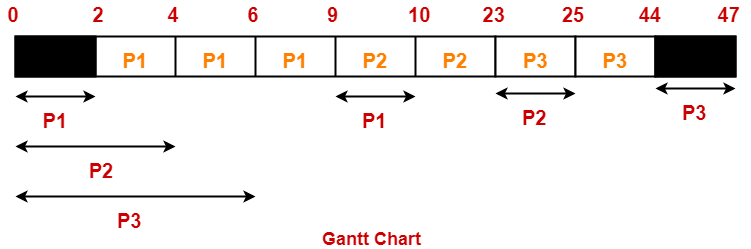Percentage of time CPU remains idle

= (5 / 47) x 100

= 10.638%

Thus, Option (B) is correct.

## Problem-02:

Consider the set of 4 processes whose arrival time and burst time are given below-

 Process No. Arrival Time Burst Time CPU Burst I/O Burst CPU Burst P1 0 3 2 2 P2 0 2 4 1 P3 2 1 3 2 P4 5 2 2 1

If the CPU scheduling policy is Shortest Remaining Time First, calculate the average waiting time and average turn around time.

## Solution-

### Gantt Chart-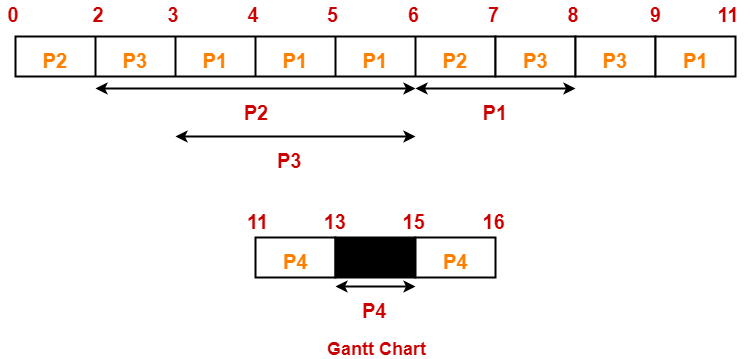Now, we know-

• Turn Around time = Exit time – Arrival time
• Waiting time = Turn Around time – Burst time

Also read- Various Times Of Process

 Process Id Exit time Turn Around time Waiting time P1 11 11 – 0 = 11 11 – (3+2) = 6 P2 7 7 – 0 = 7 7 – (2+1) = 4 P3 9 9 – 2 = 7 7 – (1+2) = 4 P4 16 16 – 5 = 11 11 – (2+1) = 8

Now,

• Average Turn Around time = (11 + 7 + 7 + 11) / 4 = 36 / 4 = 9 units
• Average waiting time = (6 + 4 + 4 + 8) / 4 = 22 / 5 = 4.4 units

## Problem-03:

Consider the set of 4 processes whose arrival time and burst time are given below-

 Process No. Arrival Time Priority Burst Time CPU Burst I/O Burst CPU Burst P1 0 2 1 5 3 P2 2 3 3 3 1 P3 3 1 2 3 1

If the CPU scheduling policy is Priority Scheduling, calculate the average waiting time and average turn around time. (Lower number means higher priority)

## Solution-

The scheduling algorithm used is Priority Scheduling.

### Gantt Chart-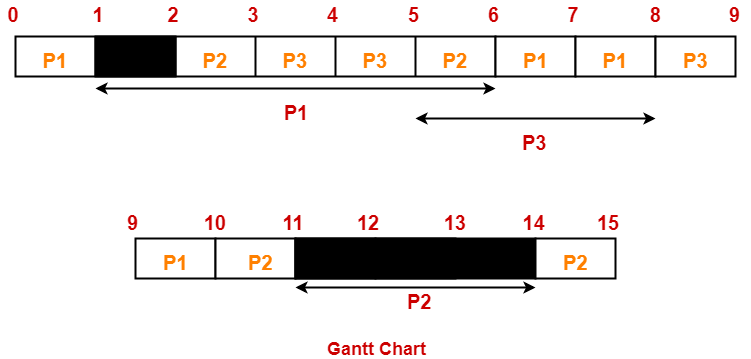Now, we know-

• Turn Around time = Exit time – Arrival time
• Waiting time = Turn Around time – Burst time

 Process Id Exit time Turn Around time Waiting time P1 10 10 – 0 = 10 10 – (1+3) = 6 P2 15 15 – 2 = 13 13 – (3+1) = 9 P3 9 9 – 3 = 6 6 – (2+1) = 3

Now,

• Average Turn Around time = (10 + 13 + 6) / 3 = 29 / 3 = 9.67 units
• Average waiting time = (6 + 9 + 3) / 3 = 18 / 3 = 6 units

Next Article- Introduction to Process Synchronization

Get more notes and other study material of Operating System.

Watch video lectures by visiting our YouTube channel LearnVidFun.

## Priority Scheduling-

In Priority Scheduling,

• Out of all the available processes, CPU is assigned to the process having the highest priority.
• In case of a tie, it is broken by FCFS Scheduling.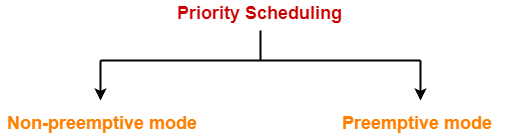• Priority Scheduling can be used in both preemptive and non-preemptive mode.

• It considers the priority of the processes and allows the important processes to run first.
• Priority scheduling in preemptive mode is best suited for real time operating system.

• Processes with lesser priority may starve for CPU.
• There is no idea of response time and waiting time.

## Important Notes-

### Note-01:

• The waiting time for the process having the highest priority will always be zero in preemptive mode.
• The waiting time for the process having the highest priority may not be zero in non-preemptive mode.

### Note-02:

Priority scheduling in preemptive and non-preemptive mode behaves exactly same under following conditions-

• The arrival time of all the processes is same
• All the processes become available

## Problem-01:

Consider the set of 5 processes whose arrival time and burst time are given below-

 Process Id Arrival time Burst time Priority P1 0 4 2 P2 1 3 3 P3 2 1 4 P4 3 5 5 P5 4 2 5

If the CPU scheduling policy is priority non-preemptive, calculate the average waiting time and average turn around time. (Higher number represents higher priority)

## Solution-

### Gantt Chart-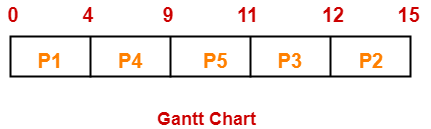Now, we know-

• Turn Around time = Exit time – Arrival time
• Waiting time = Turn Around time – Burst time

Also read- Various Times of Process

 Process Id Exit time Turn Around time Waiting time P1 4 4 – 0 = 4 4 – 4 = 0 P2 15 15 – 1 = 14 14 – 3 = 11 P3 12 12 – 2 = 10 10 – 1 = 9 P4 9 9 – 3 = 6 6 – 5 = 1 P5 11 11 – 4 = 7 7 – 2 = 5

Now,

• Average Turn Around time = (4 + 14 + 10 + 6 + 7) / 5 = 41 / 5 = 8.2 unit
• Average waiting time = (0 + 11 + 9 + 1 + 5) / 5 = 26 / 5 = 5.2 unit

## Problem-02:

Consider the set of 5 processes whose arrival time and burst time are given below-

 Process Id Arrival time Burst time Priority P1 0 4 2 P2 1 3 3 P3 2 1 4 P4 3 5 5 P5 4 2 5

If the CPU scheduling policy is priority preemptive, calculate the average waiting time and average turn around time. (Higher number represents higher priority)

## Solution-

### Gantt Chart-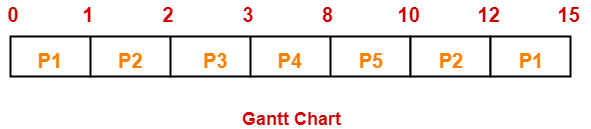Now, we know-

• Turn Around time = Exit time – Arrival time
• Waiting time = Turn Around time – Burst time

 Process Id Exit time Turn Around time Waiting time P1 15 15 – 0 = 15 15 – 4 = 11 P2 12 12 – 1 = 11 11 – 3 = 8 P3 3 3 – 2 = 1 1 – 1 = 0 P4 8 8 – 3 = 5 5 – 5 = 0 P5 10 10 – 4 = 6 6 – 2 = 4

Now,

• Average Turn Around time = (15 + 11 + 1 + 5 + 6) / 5 = 38 / 5 = 7.6 unit
• Average waiting time = (11 + 8 + 0 + 0 + 4) / 5 = 23 / 5 = 4.6 unit

To gain better understanding about Priority Scheduling,

Watch this Video Lecture

Next Article- Practice Problems On CPU Scheduling Algorithms

Get more notes and other study material of Operating System.

Watch video lectures by visiting our YouTube channel LearnVidFun.